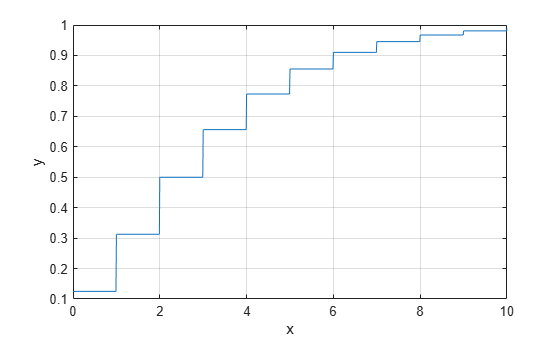nbincdf

Negative binomial cumulative distribution function

Syntax

y = nbincdf(x,R,p)
y = nbincdf(x,R,p,'upper')

Description

y = nbincdf(x,R,p) computes the negative binomial cdf at each of the values in x using the corresponding number of successes, R and probability of success in a single trial, p. x, R, and p can be vectors, matrices, or multidimensional arrays that all have the same size, which is also the size of y. A scalar input for x, R, or p is expanded to a constant array with the same dimensions as the other inputs.

y = nbincdf(x,R,p,'upper') returns the complement of the negative binomial cdf at each value in x, using an algorithm that more accurately computes the extreme upper tail probabilities.

The negative binomial cdf is

$y=F\left(x|r,p\right)=\sum _{i=0}^{x}\left(\begin{array}{c}r+i-1\\ i\end{array}\right){p}^{r}{q}^{i}{I}_{\left(0,1,...\right)}\left(i\right)$

The simplest motivation for the negative binomial is the case of successive random trials, each having a constant probability p of success. The number of extra trials you must perform in order to observe a given number R of successes has a negative binomial distribution. However, consistent with a more general interpretation of the negative binomial, nbincdf allows R to be any positive value, including nonintegers. When R is noninteger, the binomial coefficient in the definition of the cdf is replaced by the equivalent expression

$\frac{\Gamma \left(r+i\right)}{\Gamma \left(r\right)\Gamma \left(i+1\right)}$

Examples

collapse all

x = (0:15);
p = nbincdf(x,3,0.5);
stairs(x,p)